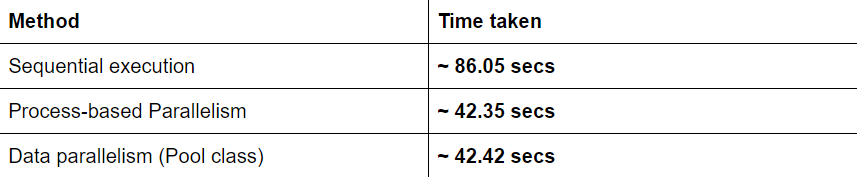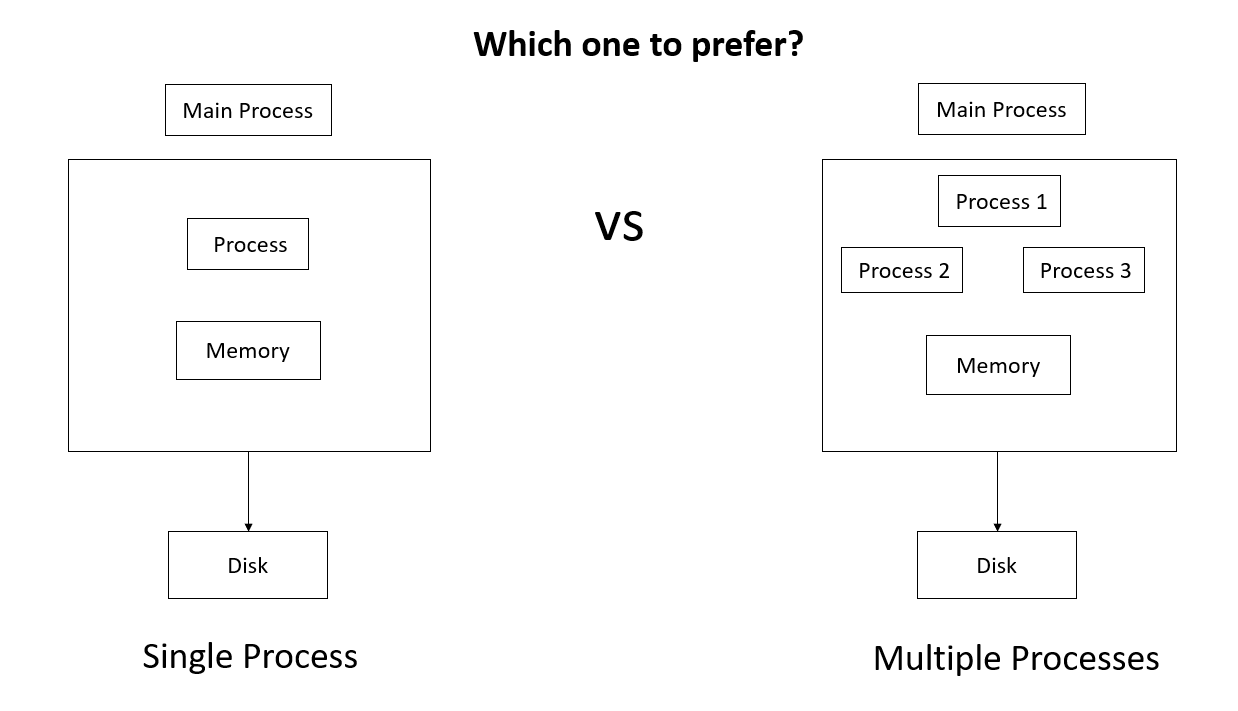# Process based parallelism in python

Parallelism is defined as the execution of tasks/processes simultaneously which helps in reducing the time taken if those tasks were to run sequentially. Now to achieve parallelism we need support from both the hardware (should have more than one core) and the software (processes must support parallelism).

For example - In a factory, there is only one supply chain where one person examines the products, now if there were to be another supply chain the time taken would have been less, this is the hardware limitation, for another instance let’s say we have two supply chain but the products are dependent on each other and can’t be examined separately, this would be the software limitation.

So today in this article, we are going to dive deep and see how process-based parallelism can be achieved in python.

### Multiprocessing- Context and Start Methods

Multiprocessing is a module that supports the spawning of processes. It uses subprocesses instead of threads thereby avoiding Global Interpreter lock (GIL) and leveraging multiple processors of a machine.

In multiprocessing, there are 3 ways to start a process namely spawn, fork and forkserver. Fork and forkserver can only be used in Unix and fork is the default for Unix while spawn can be used in Windows and macOS as well and is the default for both.

Multiprocessing module does provide a method through which we can select from the above 3 ways. Below is the code for the same

``````import multiprocessing as mp
print('Addition of two numbers is :',a+b)

if __name__ == '__main__':
mp.set_start_method('spawn')
# or set_start_method(fork/forkserver)
a = b = 2
p.start()
p.join()
``````

The `set_start_method()` chooses the spawn method to create the child process. Then a process is initialized by passing a target function(add) and the arguments(a,b) to the constructor. The target function is called when we start a process using `p.start()`.

The `set_start_method()` should only be used once in the program.

Now what if we want to use spawn, fork or forkserver in the same program, here `get_context()` comes to the rescue. It is a method which is used to get a context object which has the same API as a multiprocessing module and using those objects processes can be initialized.

``````import multiprocessing as mp
print('Addition of two numbers is :',a+b)

if __name__ == '__main__':
# using the spawn method
ctx1 = mp.get_context('spawn')
# using the fork method
ctx2 = mp.get_context('fork')
a=b=1
p1.start()
p2.start()
p1.join()
p2.join()
``````

In the above code, we are initializing two context objects of spawn and fork and using each context object a process has been started.

It may be possible that an object of one context is not compatible with the object of another context. So care must be taken while sharing objects. For eg: a `lock` created using spawn context cannot be passed to another process having a fork or forkserver context.

### Communication Between Processes

Now we have created the processes but what if they need to communicate with each other? The multiprocessing module provides 2 ways for communication among processes:

• Using Queues : `Queue` provides a simple way to communicate amongst the processes and pass the data back and forth
``````import multiprocessing as mp
def push_elements(List, q):
print('Elements pushed into queue : ',end = ' ')
for n in List:
print(n,end = ' ')
q.put(n)

def pop_elements(q):
print('\nElements popped from queue: ',end = ' ')
while not q.empty():
print(q.get(),end = ' ')

if __name__ == "__main__":
List = [5,10,15,20]
q = mp.Queue()
p1 = mp.Process(target=push_elements, args=(List, q))
p2 = mp.Process(target=pop_elements, args=(q,))
p1.start()
p1.join()
p2.start()
p2.join()
``````

In the above code, there are two processes `p1` and `p2` communicating through the Queue, `p1` is pushing the list elements from the list and `p2` is popping the elements from the queue.

• Using `Pipes` : The multiprocessing module provides the `Pipe()` function for processes to communicate. A pipe has two endpoints through which data can be sent and provides a duplex connection.
``````from multiprocessing import Process, Pipe
def sender(conn,message):
print("Message send from one process :",end = ' ')
print(message)
conn.send(message)
conn.close()

if __name__ == '__main__':
parent_conn, child_conn = Pipe()
message = "Hi! How are you"
p = Process(target=sender, args=(child_conn,message))
p.start()
print("\nMessage received at another process :",end = ' ')
p.join()
``````

In the above code, the `Pipe()` returns two connection objects. The process `p` uses one connection object to send a message and the parent process uses another connection object to receive the message.

### Process-based Parallelism

Now we shall see how the multiprocessing module helps to achieve process-based parallelism and further will compare the results. Below is the code if we do not leverage the parallelism:

``````import time
def fibonacci(n):
# function returning nth Fibonacci number
if n==1:
return 0
elif n==2:
return 1
else:
return fibonacci(n-1)+fibonacci(n-2)

if __name__ == '__main__':
start = time.time()
for i in range(1,41):
fibonacci(i)
print('Total time taken :', time.time() - start)
``````

In the above code, we are computing the Fibonacci sequence till 40. This whole program took approximately 86.05 secs to execute.

Now we shall take some help from the multiprocessing module and see if we can perform better than this.

``````import multiprocessing as mp
import time

def Fibonacci(n):
# function returning nth Fibonacci number
if n==1:
return 0
elif n==2:
return 1
else:
return Fibonacci(n-1)+Fibonacci(n-2)

def FibonacciUtil(print_lock,worker):
Fibonacci(worker)
with print_lock:
print(mp.current_process().name,worker)

def processor(print_lock,q):
# function where process pick up the job
while True:
worker = q.get()
if worker is None:
# exit from the loop if no job is left in the queue
break
FibonacciUtil(print_lock,worker)

if __name__ == '__main__':
q = mp.Queue()
print_lock = mp.Lock()

processes = []
for i in range(4):
process = mp.Process(target=processor,args=(print_lock,q))
processes.append(process)

for process in processes:
process.start()

start = time.time()
for job in range(1,41):
q.put(job)

for process in processes:
q.put(None)

for process in processes:
process.join()
print('Total time taken :',time.time() - start)

``````

The above program took approximately 42.35 secs to execute which is almost half the time it takes to run it sequentially. In the above code, we are creating a `lock` (mutex) on the printing function so that only one process should be able to print at a time otherwise there will be a mess in the printing console. Thereafter a list of processes is created (4 here) to perform the job. Now the processes are started and `processor` function is called. In the processor function all the processes will wait till we put the job in the queue, then function `fibonacci_util` will be called. In that function, we are calculating the `nth` Fibonacci number. Now let’s go back to the main function here let’s put some jobs in the queue. And finally what `process.join()` does is to tell the main process to hold it’s execution until all the processes have finished their execution.

Data parallelism using Pool Class

Multiprocessing module also introduces a `Pool` class which helps us in parallelizing the execution of a function by distributing the data across different processes. Now we will be writing some code to understand it better.

``````from multiprocessing import Pool
import time
def fibonacci(n):
# function returning nth Fibonacci number
if n==1:
return 0
elif n==2:
return 1
else:
return fibonacci(n-1)+fibonacci(n-2)

if __name__ == '__main__':
data = []
for i in range(1,41):
data.append(i)
start = time.time()
p = Pool(4)
p.map(fibonacci, data)
print('Total time taken :',time.time()-start)
``````

The time taken by the above program is approximately 42.42 secs. Here first we are storing the data to be passed to the `fibonacci` function in a list and then with the help of a `pool of 4 workers`, we are passing the data to the function parallelly since the values computed will be independent of each other.

### ComparisonUsing the process-based parallelism we are able to reduce the total time taken by more than half than sequential execution.

The values in comparison can differ depending on the system

### Process-based parallelism: Yes or NoShould process-based parallelism always be used? The answer is “NO”.The process-based parallelism is helpful when the process includes more CPU based computation than `I/O` operations(eg: time.sleep()). Because If we had more I/O operations than the process would spend most of the time sitting `idle`, for eg. waiting for an Html file to be downloaded, rather than executing in the CPU. Now since processes have a huge overhead it takes time to switch(context-switching) from CPU to the idle state and come back to CPU which adds up to the final result and that is not what we desire. So process-based parallelism is preferred when we are dealing with some CPU intensive tasks.

### Conclusion

Python achieves parallelism with the help of the Multiprocessing module and the above results show that we can gain a lot in terms of computation time but there are some downfalls also. Although the Multiprocessing module helps in reducing the computation time it increases the complexity of the code and as a result debugging becomes difficult. So care must be taken while creating and handling multiple processes.

# Akshay Malav

Final Year CSE Undergrad at IIT Jodhpur | Interned at Mathworks

Akshay has published 1 article so far